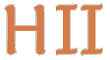# Hilbert II

 English Deutsch
 introduction news mathematics QEDEQ planning download glossary development prototype links contact site map
Mathematics

### Logic

In Hilbert II a formal language is used which enables us to describe most domains of mathematics. It is a first order predicate calculus based on the text Elements of Mathematical Logic from P. S. Novikov. The logical axioms and basic rules originate from the book Principles of Mathematical Logic (Grundzüge der theoretischen Logik) (1928) by D. Hilbert and W. Ackermann.

An overview about the used logic in Hilbert II can be found in the script Elements of Mathematical Logic. There are all rules, axioms, definitions and propositions assembled. The propositions are not complete and currently proofs are missing, but the mathematical orientation becomes clear. Meanwhile we have a script with the axioms and inference rule of first-order predicate calculus which contains the beginning propositions of propositional calculus inclusive formal proofs: see qedeq_formal_logic_v1.

### Set Theory

Beside logical ones the only axioms in Hilbert II are those of axiomatic set theory. As usual for mathematics the axioms of all other theories could be expressed as simple predicate constant definitions. With common mathematical practice in mind, the set theory used here is not ZFC but Morse-Kelley (an impredicative Neumann-Bernays-Gödel extension). Our mathematical basis text is E. J. Lemmon's wonderful Introduction to Axiomatic Set Theory.

In Axiomatic Set Theory we construct systematically our set theory. This is a living document and is updated from time to time. Especially at the locations marked with "+++" additions or changes will be made. See also under planning.

### Literature

• D. Hilbert und W. Ackermann, Grundzüge der theoretischen Logik, Berlin: Springer, 1928.
• P. S. Novikov, Elements of Mathematical Logic, Edinburgh: Oliver and Boyd, 1964.
• A. N. Whitehead und B. Russell, Principia Mathematica, London: Cambridge University Press, 1910.
• E. J. Lemmon, Introduction to Axiomatic Set Theory, London: Routledge & Kegan Paul Ltd, 1968.
• J. Schmidt, Mengenlehre I, Mannheim: BI, 1966.
• J. D. Monk, Introduction to Set Theory, New York: McGraw-Hill, 1996.
• G. Takenti, W. M. Zaring, Introduction to Axiomatic Set Theory, New York: Springer, 1971.

update 2014-01-20 09:20:48+0100